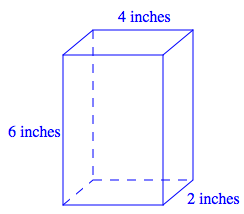### Home > MC1 > Chapter 9 > Lesson 9.3.4 > Problem9-159

9-159.

Draw a diagram of a box with a height of $6$ in., a width of $4$ in., and a depth of $2$ in. and then calculate its volume. Show all of your work.

Below is one diagram you could draw for this problem. Can you calculate the volume? Remember to show your work.